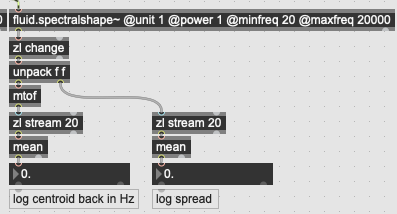# Spectralshape~ @unit 1 question

The @unit 1 outputs log frequencies as floating point MIDI if I understand correctly.
In the help patch under ‘logarithmic scale’ I see that on the frequency output ‘mtof’ is used, which makes sense. The spread is also expressed in Hz, but no mtof is used?What is the reasoning behind? Thanks.

because both are in semitone. the first has a reference (69 = 440) but the second hasn’t: it is a range. So if you got 60 10 as those 2 values it would be +/- 10 semitones around 60 to describe the weighted mean and its standard deviation, or the peak of the gaussian curve and its width.

thanks